Session 3

COMPUTATIONAL AND MATHEMATICAL METHODS I

Chairman: R. Cotta

NUMERICAL HEAT TRANSFER MODULE ON INTERNET

Tien-Mo Shih* and W. J. Minkowycz**

* Department of Mechanical Engineering, University of Maryland,

College Park, MD 20742, USA

** Department of Mechanical Engineering, University of Illinois,

Chicago, IL 60607, USA

It is proposed in this article that the numerical heat transfer (NHT) community consider the possibility of agreeing to adopt an NHT module. Such a module basically computes the discretized equations governing conservation of mass, momentum, energy, and species at an arbitrary interior grid node. It will be available on the internet for any user to retrieve.

Using this module, a heat transfer researcher can spend a relatively small amount of his time in prescribing the boundary conditions of his physical problem, and in developing his own computer code.

Taking this opportunity offered by ICHMT, we would like to raise the following key questions:

1. What is the main difference between the proposed NHT module and an NHT computer code?

2. Is it beneficial for the NHT community to establish a center where

• a module is provided, without charge (or a small charge), for anyone who wishes to retrieve it?
• a few full-time consultants are available to answer technical questions asked by the users?
• a few full-time applied mathematicians and NHT engineers continue to do research to improve the module?

3. If the answer to question #2 is positive, is it safe to assume that there may not be any hidden major technical difficulties?

4. If the answer to #3 is also positive, then at what technical level should this module be written to meet the community's current/future need?

We will offer our own opinions tentatively, and hope that our questions and answers will stimulate further discussions. We then plan to edit the feedbacks, and publish the result at a later date.

AN AUGMENTED LAGRANGIAN METHOD FOR COMPUTING BINGHAM FLUID FLOWS

L. Boscardin*, JC. Latche* and P. Lesaint**

13108 St Paul-Lez-Durance CEDEX, France

**Laboratoire de calcul scientifique - Université de Franche-Comté

16, route de Gray -25000 Besançon - France

The aim of this paper is to present a finite element method to solve the 2D Navier-Stokes equations for a Bingham fluid flow.

In a first part, we present the mathematical approach based on an Augmented Lagrangian Method. The next section is devoted to the description of two different algorithms, based respectively on Uzawa and Newton-GMRES technique, to solve the obtained discrete system. Finally, the performances of these algorithms are compared on the benchmark problem of the driven lid cavity.

Mathematical approach to establish the discrete system

The global strategy we use to solve the Navier-Stokes problem is the following1-2: the momentum balance equation is discretized in time by splitting the operator in its convection and diffusion part. Over each time step, the convection equation is solved by a forward characteristic technique. The obtained velocity is then taken as initial condition for the diffusion problem, which is solved by a finite element method.

The motion equations for a Bingham fluid differ from the Newtonian ones by the expression of the shear stress which is only used in the diffusion step. That is the reason why, in the following of this paper, we restrict our attention to this problem, namely the generalized Stokes problem.

It can be shown3 that the weak formulation of this partial derivative equation leads, for the case of a Bingham fluid, to a variational inequality, which may be formulated as a minimization problem of the form :whereis a nonlinear functional which is nondifferentiable at each point where the strain rate is equal to zero.

A solution to this problem has already been proposed by Bercovier4 who used a penalty approach to deal with the uncompressibility constraint and a regularization technique to cope with the nondifferentiability of the functional. The method presented here is based on radically different choices: the uncompressibility constraint is taken into account by a duality type argument and the nondifferentiability problem is overcome applying the decomposition-coordination technique introduced by Fortin and Glowinski5.

Let us introduce the Lagrangian functional where the uncompressibility constraint is imposed through a Lagrange multiplier (denoted q).

The minimization problem (1) is now set under the form of the saddle point problem :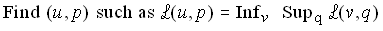with :Because,is not differentiable with respect to the variable v, we cannot, as usually, derive from the above expression the equivalent set of normal equations.

We then introduce a new variable z defined as the strain rate D(v) associated to the velocity field v, and reformulate the problem as :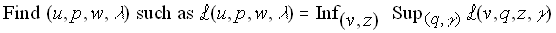with :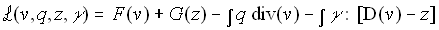The functionals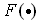and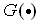are chosen in such a way that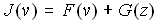andis differentiable.

As said before, the optimality condition for w cannot be traduced by a normal equation. Nevertheless, if we approximate this variable using a P0 element (which is possible because w stands for derivatives of the velocity and the required regularity is then only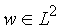), the obtained discrete optimization problem degenerates into a family of scalar problems (one per mesh) whose solution can be given explicitly, as a nonlinear combination of the other three variables.

In order to take advantage of this property, the discretization of the equations has been performed using Q1 element for the velocity and P0 elements for p, w and l. It is known that this approximation may lead to stability problems6. This choice was motived by the fact that our interest is focused on the velocity and that this element has a low cost. Nevertheless, in the near future, higher degree approximations will be implemented.

We have then obtained finally a set of nonlinear equations. The development of numerical algorithms to solve this system will be the object of the next section.

Solution algorithms

In this part, we describe two different methods to solve the discret system.

The classical method is to use a Uzawa algorithm with a relaxation technique to solve at each iteration the minimization problem. In order to enhance the convergence of the method, the discrete Lagrangian is augmented with respect to both constraints. This algorithm is described in reference 5 (algorithm referred to as ALG1).

The second method we propose is to apply to the nonlinear system a Newton algorithm. We then write the system to solve as. At each iteration, a linear system involving the Jacobian matrix of the functional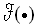must be solved : this is performed using a Krylov method (in our case GMRES), well suited to large sparse matrix.

We then obtain the following algorithm :

Set X0

From n = 0 until convergence, do :

- Solve- Set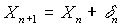Numerical results

In order to compare the performance of the previous algorithms, we have chosen as benchmark problem the flow of a Bingham fluid in a driven lid cavity. The computation was carried out for a flow of Re =1000 with a yield stress g =10 Pa corresponding to a Bingham number of 10. We recall that the Bingham number is defined as :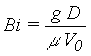where D and V0 are respectively the characteristic length and the velocity and that it can be interpreted as the ratio of the "plastic" stresses to the viscous ones.

The computation was performed with two different meshings : one of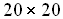square elements and a more refined non-uniform one.

The initial velocity field was chosen to be the solution of the Stokes problem and the transient was continued until obtaining the steady solution of the Navier-Stokes equations. As classically obtained for the Stokes problem4 , we observed two plugs regions : one moving at the center of the vortex and one motionless in the bottom of the cavity. For the Stokes problem, the flow admits the vertical line at the center of the cavity as symmetry axis. Results show a good convergence with respect to the spatial discretization.

For the Uzawa algorithm, the CPU time is about five times more important than for a Newtonian fluid. A comparison with the second algorithm described below (Newton-GMRES method) will be performed in the near future and provided in the full paper.

REFERENCES

1. Benque J.P., Ibler B., Keramsi A., Labadie G. (1980), A finite Element Method for the Navier Stokes equations, Proceedings of the third international conference on finite elements in flow problems, Banff. Alberta, Canada, 10-13 June 1980
2. Pironneau O., On the transport diffusion algorithm and its applications to the Navier Stokes equations, Numer. Math., Vol 38, pp 309-332, 1982.
3. Duvaut G. and Lions J.L., Les inéquations en mécanique et en physique, Dunod, Paris, 1972.
4. Bercovier M. and Engelman M., A finite Element Method for Incompressible Non-Newtonian Flows, J. Comput. Phys., Vol 36, pp 313-326, 1980.
5. Fortin M. and Glowinski R., Méthodes de Lagrangien augmenté, Dunod, Paris, 1982.
6. Brezzi F. and Fortin M., Mixed and Hybrid Finite Element Methods, Springer-Verlag, New-York, 1991.

EFFICIENT NUMERICAL CALCULATION OF UNSTEADY FREE CONVECTION

G. de Vahl Davis, E. Leonardi and V. Timchenko

School of Mechanical and Manufacturing Engineering, University of NSW

and

M. Wolfshtein

Current position: Technion, Haifa

INTRODUCTION

Unsteady free convection became a popular problem in recent years due to the interest in g-jitter. The flow generated by buoyancy forces is usually slow, and consequently laminar. The temperature differences are not high either, permitting the assumption of nearly uniform density and viscosity. Therefore it is possible to use the incompressible Navier-Stokes equations to describe the phenomenon. Indeed the only part of the equations where density changes have to be taken into account is the body force due to buoyancy. This term is simplified using the Bousinesq approximation which neglects the influence of pressure on the density, and linearizes the influence of temperature. Consequently it is possible to use existing computer codes for incompressible viscous flow problems to solve many free convection problems. Many of these codes are designed to solve steady state problems. However, such codes can be easily adapted to unsteady situations, with minor modifications. In general the solution of unsteady flow problems requires large processor time, due to the need to solve one or more Poisson equations at every time step. Thus it is highly desirable to use efficient Poisson solvers when attempting to solve an unsteady problem. The classical solution to this problem is by using advanced algorithms (e.g. spectral or multi-grid). However, such algorithms are not always easy to use, and their efficiency may deteriorate when the problem considered is complicated either geometrically or physically.

In this paper we consider spatial discretization methods such as finite difference or finite volumes. Our approach to the problem is based on the fact the Poisson Equations we have to solve are often identical in all respects but the r.h.s. (the so called source) term. As the rate of convergence of a Poisson solver depends on the properties of the l.h.s. of the equation, it appears that we can actually utilize this fact to enable an efficient solution of the Poison equations. The basic idea is to determine the optimal numerical parameters of the solution method by numerical experimentation before the computation starts, and then to use them all along the solution, in all time steps.

It is our aim to use simple methods which are easy to program and run. Therefore we did not consider the more advanced multi-grid or spectral methods. Thus the methods considered here are three: Successive Over Relaxation (SOR), Alternating Directions Implicit (ADI), and Conjugate Gradient (CG). All of these methods are easily incorporated in existing codes. SOR methods require an optimal over-relaxation parameter. ADI requires an optimal time step. Either of these two parameters can be determined by numerical experimentation, and as long as the mesh geometry is kept unchanged the parameters can remain unchanged as well. Thus a limited number of numerical experiments is required initially to determine these values. Once determined they do not need any modification.

Most numerical schemes for the incompressible Navier-Stokes equations require the solution of one or more Poisson equations, but the boundary conditions required may vary between different Navier-Stokes solvers. The present project was undertaken in the context of a vorticity-vector potential formulation of the Navier-Stokes equations. Therefore the Poisson equations used in this paper have different boundary conditions from the equations used with primitive variables methods. Yet we believe that the conclusions to be drawn are more general and may be applicable to other solvers as well.

The approach used to study this problem was by actually performing the numerical experiments outlined above on all three methods. Three dimensional problems were considered, and results were obtained for various meshes.

The present formulation is based on the 3 equations for the three components of the vorticity. The pressure has been eliminated in the process, but the velocities are still required. They are computed from the vector potential which is governed by 3 Poisson equations. The velocities are computed by straight forward differentiation of the vector potential.

Boundary conditions for the vorticity equations are the usual ones. The boundary condition for the vector potential is different on different sides. When the normal to a face is in the direction of the component of the vector potential a zero gradient condition is applied. On other faces a zero value condition is applied.

As discussed above, we deal in the present paper with the solution of the Poisson equation only.

The solutions are performed on a non staggered finite difference mesh. The Douglas ADI marching scheme is applied to the vorticity equations. The Poisson equations for the vector potential are solved using SOR, ADI, and CG as discussed in the introduction above.

The iterative successive over-relaxation method requires an over-relaxation parameter w which should always be smaller than 2. When w =1 the method is reduced to the Gauss-Seidell method, and when w <1 it is called under-relaxation. The parameter w can be analytically determined only for simple cases. However, as it depends only on the coefficients of the finite difference equation, it can be determined in advance by trial and error, and it does not change during time marching whenever the coefficients of the finite difference equation remains constant. This is the case in many calculations.

The ADI method used here was proposed by Douglas in the US and by Samarskii and Andreev in the former Soviet Union. The scheme is iterative, and it is unconditionally stable, thus enabling long time steps. However, the minimum number of iterations is reached at a certain given time step which has to be found by trial and error as well. It is common to relate the time step to the spatial step by the quantity s=D t/D x2. Thus we seek the minimal s. The Conjugate Gradient method is iterative as well. However, it does not contain a free numerical parameter.

The performance (measured in iterations until convergence) of the three methods was found to depend on various parameters, like the number of mesh points, the aspect ratio of the computational domain, and the boundary conditions. As explained above, a zero gradient boundary condition is used in one direction (parallel to the appropriate component of the vector potential), and a zero value boundary condition is used in the other two directions. In the tests we did not change the directions, but we changed the number of mesh points and the aspect ratio in order to cover all possible cases. Thus we applied the zero gradient boundary condition in the z direction and the zero value in the x and y directions. We also used the same number of mesh pints in the x and y directions. In all cases a problem with an exact solution was used. The initial condition was always 90% of the exact solution. Convergence was assumed when the difference between consecutive values was smaller than 10-8. All runs were performed on a Pentium processor, and the number of mesh points varied between 11 and 41 in each direction. The goal was to find the optimal values of the parameter w (for the SOR method) and the parameter s (for the ADI method). Then the best performance of both methods was compared with the CG method. Two criteria for efficiency were recorded, namely the number of iterations and the processor time.

The results of all computations are summarized in the following table. In this tables nx, ny and nz are the number of mesh points in the x, y and z direction; omega is the SOR coefficient (equal to unity when no over relaxation is used), s is the ratio of the time step to the spatial step squared, noit is the number of time steps or iterations, and CPU is the processor time on a PENTIUM computer. In all these runs the number of mesh points in the x and y direction was equal, and arz defines the aspect ratio of the computational domain.

 ADI SOR CG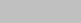nx,ny nz arz s noit cpu w noit cpu noit cpu 11 11 1 10 9 0.05 1.6 31 0.08 33 0.05 21 21 1 30 8 0.2 1.78 61 0.62 53 0.28 31 31 1 65 8 0.69 1.84 88 3.21 77 1.17 41 41 1 120 8 1.62 1.88 115 10.39 87 3.74 31 41 1.33 110 10 1.14 1.86 99 5.04 91 2.22 21 31 1.5 55 12 0.46 1.81 70 1.14 63 0.48 11 21 2 20 17 0.12 1.7 41 0.16 44 0.1 21 41 2 85 16 0.8 1.82 74 1.65 86 0.86 11 31 3 25 27 0.27 1.75 53 0.27 48 0.14 11 41 4 35 38 0.49 1.7 50 0.33 61 0.22

The results indicate that the ADI method is generally superior to the other two both in processor time and in number of iterations. Moreover, it appears that the ADI method does not need more time steps for larger meshes or high aspect ratios, while the two other methods require more iterations or time steps.

A NODAL INTEGRAL METHOD FOR MOVING BOUNDARY PHASE CHANGE PROBLEMS

Rizwan-uddin
Department of Nuclear Engineering, and
Computational Science and Engineering
University of Illinois at Urbana Champaign
214 NEL,103 S. Goodwin Ave.
Urbana, IL 61801

Most traditional numerical techniques are known to have difficulties when applied to the Stefan problem with time-dependent applied surface conditions1,2. We here report the development of a nodal integral method (NIM) to solve the one-dimensional Stefan problem with time-dependent boundary conditions. NIMs have been developed over the last two decades and applied to problems ranging from the neutron diffusion equation to the Navier-Stokes equations3. Recent modification to the NIM, when applied to the nonlinear Burgers equation, showed even better results than the conventional NIM4.

Two key steps in the development of the NIM for any problem are,

1. the transverse integration processthrough which the PDE(s) is reduced to an ODE for dependent variables locally averaged (over the node) in all other directions,
2. the analytical solution of as much of these ODEs as possible, approximately treating the terms that cannot be handled exactly.
Hence, a PDE in two independent variables is reduced to two ODEs. These ODEs are solved locally over computational nodes. Because of the built-in analyticity in the resulting computational scheme, these methods have been highly efficient in solving PDEs.

We solve the coordinate transformed Stefan problem in a fixed domain (0 < x < I) for the temperature distribution in a liquid. The governing equations arelsubject to the boundary conditions

for t < 0: R = 0
for t > 0: T = 1 at x = 0, T = 0 at x = 1

where x = x/R, and R is the position of the moving boundary made dimensionless using a characteristic length. The spatial domain is divided into nodes of size 2a, and the time step is 2. A spatial coordinate system local to each node with origin at the left edge is defined as 0 < x' < 2a . All solutions below are in local coordinates though we have dropped the primes. We treat Eq. (2) first. Note that Eq. (2) is an ODE that involves the spatial derivative of the temperature at the right edge of the domain. Equation (2) is integrated over a time step by simply assuming the derivative term on the RHS to be equal to the average value of the derivative of the temperature over that time step, which is equal to the derivative of the average temperature over the time step,We now turn to Eq. ( 1 ), defining the space and time-averaged node temperatures asOperating with, Eq. ( 1 ) becomeswhere the RHS (usually called the pseudo source term) represents the term with the time derivative averaged over the time step. In the spirit of NIM, we expand the unknown RHS, say in Legendre polynomial, truncate at an appropriate order (zeroth order here), and then solve the resulting ODE locally over the node. The solution in node i written in terms of the values at the left and right surfaces of node i, is of the formFor the time-averaged, space-dependent temperature, the average heat flux at the interface of nodes will be equated to determine the set of discretized equations. For this purpose, the temperature distribution is written out in two generic neighboring nodes; node i and node (i+1), in terms of their respective edge values. The heat flux on either side of the common interface is then evaluated and equated. The resulting equation is symbolically written asThe equation for the space-averaged, time-dependent temperature is obtained by operating Eq. ( 1 ) byThis equation is solved after substituting the expression for R(t) from Eq. (3), and the solution is of the formThe last equation can be evaluated at t = 2, to find an expression for the node averaged temperature at the next time step.

At this stage we have one equation for the position of the moving boundary, Eq. (3). We also have one equation for the space-averaged temperature of the node at the end of the time step, Eq. (8), and an equation for the time-averaged temperature at the edges of each node, Eq. (6). But, with the introduction of the pseudo source terms, Sx, St, we actually have two additional unknowns per node. The additional equations for these unknowns are obtained by ensuring that the PDE is satisfied over the space-time node in an integral sense, and by requiring the uniqueness of the node averaged temperature, independent of the order of integration. The two pseudo source terms are simply eliminated from the set of equations, leaving a set of two equations per node for the two unknowns per node, and one equation for the position of the moving boundary. The resulting set of equations are solved iteratively for each time step.

Table 1 shows the comparison of results for Ste = 0.2 obtained using the scheme described above with those reported in Ref. 2 obtained using the finite difference scheme. Here we have used only 10 nodes in the spatial direction ( 0 < x < 1 ) and the time step is 0.01. Assuming the FD results are exact, the error in the position of the moving boundary is about 1 %.

 Time, t R(t) Interpolated R(t) Finite Diff. R(t)2 0.1000 0.1904 0.1068 0.1967 0.2000 0.1100 0.1996 0.2400 0.2930 0.2441 0.2954 0.3000 0.2500 0.2998 0.4400 0.3930 0.4425 0.3941 0.4000 0.4500 0.3972 0.7000 0.4897 0.7094 0.4928 0.5000 0.7100 0.4929 1.050 0.5900 1.057 0.5913 0.6000 1.060 0.5923 1.500 0.6891 1.508 0.6906 0.7000 1.510 0.6910 2.110 0.7908 2.103 0.7912 0.8000 2.120 0.7922 2.950 0.8892 2.951 0.8893 0.9000 2.960 0.8901

Table 1

REFERENCES

1. L.S. Yao and J. Prusa, "Melting and Freezing," Adv. In Heat Transfer,19,1-95 (1989).
2. J. Menning and M.N. Özisik, Coupled Integral Equation Approach for Solving Melting or Solidification, Int. J. Heat Mass Transfer, 28, 8,1481-ï485 (1985).
3. Y.Y. Azmy and J.J. Dorning, A Nodal Integral Approach to the Numerical Solution of Partial Differential Equations, in Advances in Reactor Computations, Vol. II, pages 893-909, American Nuclear Society, LaGrange Park, IL,1983.
4. Rizwan-uddin, An Improved Coarse-Mesh nodal Integral Method for Partial Differential Equations, Num. Methods for Partial Differential Equations,13,113-145 (1997).